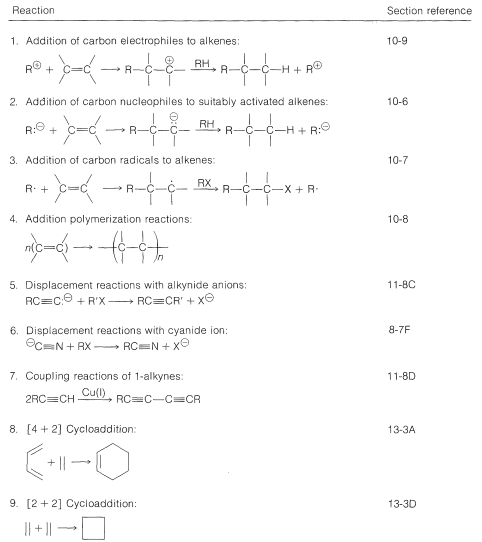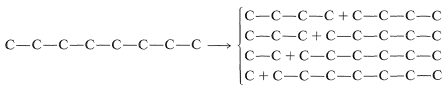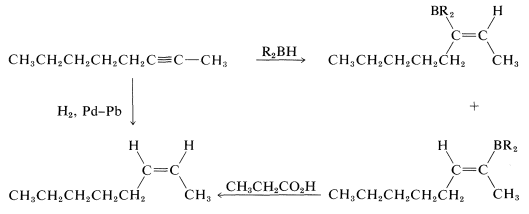# 13.7: Building the Carbon Skeleton

$$\newcommand{\vecs}{\overset { \rightharpoonup} {\mathbf{#1}} }$$ $$\newcommand{\vecd}{\overset{-\!-\!\rightharpoonup}{\vphantom{a}\smash {#1}}}$$$$\newcommand{\id}{\mathrm{id}}$$ $$\newcommand{\Span}{\mathrm{span}}$$ $$\newcommand{\kernel}{\mathrm{null}\,}$$ $$\newcommand{\range}{\mathrm{range}\,}$$ $$\newcommand{\RealPart}{\mathrm{Re}}$$ $$\newcommand{\ImaginaryPart}{\mathrm{Im}}$$ $$\newcommand{\Argument}{\mathrm{Arg}}$$ $$\newcommand{\norm}{\| #1 \|}$$ $$\newcommand{\inner}{\langle #1, #2 \rangle}$$ $$\newcommand{\Span}{\mathrm{span}}$$ $$\newcommand{\id}{\mathrm{id}}$$ $$\newcommand{\Span}{\mathrm{span}}$$ $$\newcommand{\kernel}{\mathrm{null}\,}$$ $$\newcommand{\range}{\mathrm{range}\,}$$ $$\newcommand{\RealPart}{\mathrm{Re}}$$ $$\newcommand{\ImaginaryPart}{\mathrm{Im}}$$ $$\newcommand{\Argument}{\mathrm{Arg}}$$ $$\newcommand{\norm}{\| #1 \|}$$ $$\newcommand{\inner}{\langle #1, #2 \rangle}$$ $$\newcommand{\Span}{\mathrm{span}}$$$$\newcommand{\AA}{\unicode[.8,0]{x212B}}$$

According to the suggested approach to planning a synthesis, the primary consideration is how to construct the target carbon skeleton starting with smaller molecules (or, alternatively, to reconstruct an existing skeleton). Construction of a skeleton from smaller molecules almost always will involve formation of carbon-carbon bonds. Up to this point we have discussed only a few reactions in which carbon-carbon bonds are formed and these are summarized in Table 13-4. Other important reactions that can be used to enlarge a carbon framework will be discussed in later chapters.

Table 13-4: Some Carbon-Carbon Bond-Forming Reactions with Section ReferenceThe most logical approach to planning the synthesis of a particular carbon framework requires that one work backward by mentally fragmenting the molecule into smaller pieces that can be “rejoined” by known $$\ce{C-C}$$ bond-forming reactions. The first set of pieces in turn is broken into smaller pieces, and the mental fragmentation procedure is repeated until the pieces correspond to the carbon skeletons of readily available compounds. There almost always will be several different backward routes, and each is examined for its potential to put the desired functional groups at their proper locations. In almost all cases it is important to use reactions that will lead to pure compounds without having to separate substances with similar physical properties.

## Example

A typical synthesis problem would be to devise a preparation of cis-2-octene, given the restrictions that the starting materials have fewer than eight carbons, and that we use the $$\ce{C-C}$$ bond-forming reactions we have discussed up to now. The reasoning involved in devising an appropriate synthesis with the given restrictions will be outlined for this example in detail.

First, we can see that the carbon skeleton of the desired product can be divided to give the following combinations of fragments:Next, we have to decide what reaction or reactions would be useful to put these fragments together to reform the $$\ce{C_8}$$ chain. If we look at the list of available reactions in Table 13-4$$^4$$ for $$\ce{C-C}$$ bond formation, we can rule out 1, 2, 4, 8, and 9: 1 and 4 because the reactions are unsuitable for making unbranched chains; 8 and 9 because they make rings, not chains; and 2 because it does not work well in the absence of activating groups. Reaction 3 could be used to combine $$\ce{C_1}$$ and $$\ce{C_7}$$ units to give $$\ce{C_8}$$, as by the radical addition of $$\ce{CBrCl_3}$$ to 1-heptene:Reactions 6 and 7 could also be used to make $$\ce{C_8}$$, 6 by linking $$\ce{C_7}$$ to $$\ce{C_1}$$ and 7 by putting together two $$\ce{C_4}$$ units.$$^5$$Reaction 5 could be useful for all of the possible ways of dividing $$\ce{C_8}$$. Some of the possible combinations are:This does not exhaust the possibilities because, as 5b and 5e show, Reaction 5 can be used to make the same $$\ce{C_8}$$ compound from different sets of starting materials.

We now have to consider how to convert the $$\ce{C_8}$$ materials that we might make into cis-2-octene. The possibilities are:Of these, d is the obvious choice for first consideration because it has its functionality, a single triple bond, between the same two carbons we wish to have joined by a cis double bond in the product. Now, we have to ask if there are reactions that will convert $$\ce{-C \equiv C}-$$ to cis-. Two possibilities were mentioned previously - hydrogenation of a triple bond with the Lindlar catalyst (Section 11-2B) and hydroboration followed by treatment with propanoic acid (Section 11-6D):Either of these two reactions provides a simple and straightforward way of converting 2-octyne to cis-2-octene, so a satisfactory answer to the original problem isYou can see that even with having available only seven $$\ce{C-C}$$ bond-forming reactions and two ways of converting $$\ce{-C \equiv C}- \rightarrow$$, considerable amount of logical screening is required to eliminate unsuitable possibilities. The skilled practitioner makes this kind of diagnosis quickly in his head; at the outset you will find it useful to write the steps in your screening in the same way as we have done for this example.

$$^4$$You may wish to review the sections cited for each reaction to be sure you understand the judgments we make here as to the suitability of particular reactions for the purpose at hand.

$$^5$$Reaction 7 could also be used to make $$\ce{C_8}$$ by other combinations, such as of $$\ce{C_5}$$ and $$\ce{C_3}$$, but these would give undesirable mixtures of products. Thus, $$\ce{C-C-C-C \equiv CH} + \ce{HC \equiv C-C} \rightarrow \ce{C-C-C-C \equiv C-C \equiv C-C} + \ce{C-C \equiv.C-C \equiv C-C} + \ce{C-C-C-C \equiv C-C \equiv C-C-C-C}$$